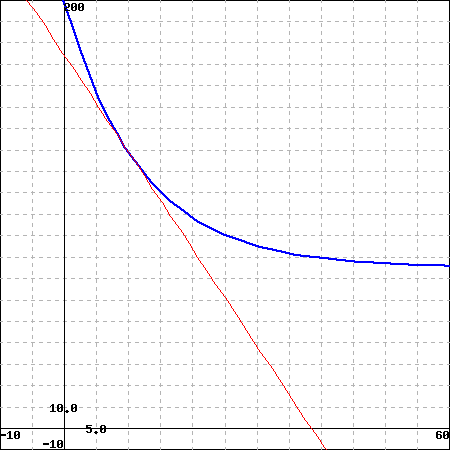According to $\>Newton's\> Law\> of\> Cooling\>$, the rate of change of an object's temperature is proportional to the difference between the temperature of the the object and that of the surrounding medium. The accompanying figure shows the graph of the temperature $\>T\>$ (in degrees Fahrenheit) versus time $\>t\>$(in minutes) for a cup of coffee, with initial temperature 200 degrees Fahrenheit, that is allowed to cool in a room with a constant temperature of 75 degrees Fahrenheit.Click on the image to see a larger graph.

(a) Estimate $\>T\>$ when $\>t = 10\>$ minutes:

(b) Estimate $\>dT/dt\>$ when $\>t = 10\>$ minutes:

Newton's Law of Cooling can be expressed as $\>\frac {dT} {dt} = k(T-T_{0})\>$,where $\>k\>$ is the constant of proportionality and $\>T_{0}\>$ is the temperature of the surrounding medium.

(c) Use the results of parts (a) and (b) to estimate the value of $\>k$.

Value of $\>k$:

You can earn partial credit on this problem.